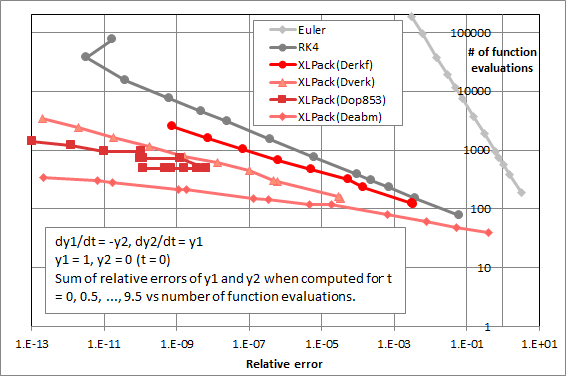# XLPack

XLPack is a set of expansion modules of Excel for numerical calculation which is developed for easy calculations on office/home PCs. It adds the features useful for wide range of scientific fields to Excel.
Excel is a widely used spreadsheet software. It is possible to program with VBA* and display the result in understandable visual form by graph functions. It can become an easy-to-use and powerful numerical calculation tool by enhancing the numerical calculation functions. (* Visual Basic for Applications: Built-in macro language of Excel)

### Software contents

XLPack consists of worksheet function library, solver and VBA subroutine/function library. These can be divided into two types: tools for numerical calculations without programming and tools that support programming.

#### Numerical calculation without programming

##### Worksheet function library

These functions can be entered directly in the Excel worksheet. Just by inputting the data into a worksheet, you can you can calculate linear equations, eigenvalues, special functions, etc.

Example of worksheet functionUsing the formulas entered in the Excel worksheet, solutions for nonlinear equations, quadrature and ordinary differential equations can be obtained by menu operation.#### Supporting VBA numerical programming

##### VBA subroutine/function library

A variety of subroutines and functions which can be called from Excel VBA are provided. By using these subroutines and functions, advanced numerical application programs can be developed in a short development period. Professional knowledge on numerical calculation is not required.

### Performance of XLPack

#### Fast computation

XLPack computes faster than the equivalent VBA program. In XLPack 6.1, the Intel oneMKL library is incorporated in linear calculations for further speeding up. The next figure shows the measurements of CPU time to solve systems of linear equations and symmetric eigen problem. In this example, for simultaneous linear equations, XLPack 6.0 is about 170 times faster than the VBA program, and XLPack 6.1 is about 10 times faster than XLPack 6.0. For eigen problem, XLPack 6.1 is about 6 times faster than XLPack 6.0.

CPU time of linear calculations#### Efficient algorithms

XLPack employs the latest efficient algorithms. The following figure shows the example of an initial value problem of ODE (ordinary differential equation). The (logarithm of) number of function evaluations is plotted against the relative error of the solution. The number of function evaluations required by the algorithms used in XLPack are less than those required by commonly used Euler method and 4th order Runge-Kutta method.

Computation amount of ordinary differential equations### Use cases

• Calculation tool for researchers and engineers
• Provides easy and practically fast numerical calculations for medium and small scale problems
• Researchers and engineers can concentrate on their main job without spending time for the details of calculation
• Development support tool
• Used for prototyping and one-time programs
• Platform in education fields
• Used for study and training of numerical calculation

## XLPack Version 6.1

### Software requirements

• Windows 10 (Version 2004 or later) or Windows 11
• Excel for Office 365, Excel 2021, Excel 2019, Excel 2016 or Excel 2013 SP1

### Features

You can use 27 major worksheet functions and 138 major VBA subroutines/functions in the basic function package. It is sufficiently powerful for normal calculations.

In addition, the introduction of four extension modules (Modules 1 – 4) will add more advanced features, and then a total of 148 worksheet functions and 902 VBA functions/subroutines can be used.

The detail list of functions is as follows.

XLPack detail list of functions

The orange parts are provided as basic functions.

### Products

• XLPack Basic (basic functions)
• Basic function package with major features. Same software requirements and performance as full version
• It can be installed as “XLPack Basic” from Microsoft Store free of charge.
• Additional functions are divided into four modules – M1: Linear computation (real), M2: Linear computation (complex), M3: Special functions, nonlinear computation, M4: Interpolation, differential/integral equations, random numbers (see more details in the detail list of functions above)
• It can be installed as “XLPack Addons” from Microsoft Store, and each module can be purchased by in-app purchase
• Manuals and sample worksheets are available online

For the detail installation and setup procedures, see XLPack 6.1 User’s Guide.

### XLPack API

Calculation modules of XLPack can be called through this interface as the numerical library from other languages such as C/C++, Python, Julia, C#, F#, VB.NET and Pascal.

Refer to XLPack API Guide for more details.